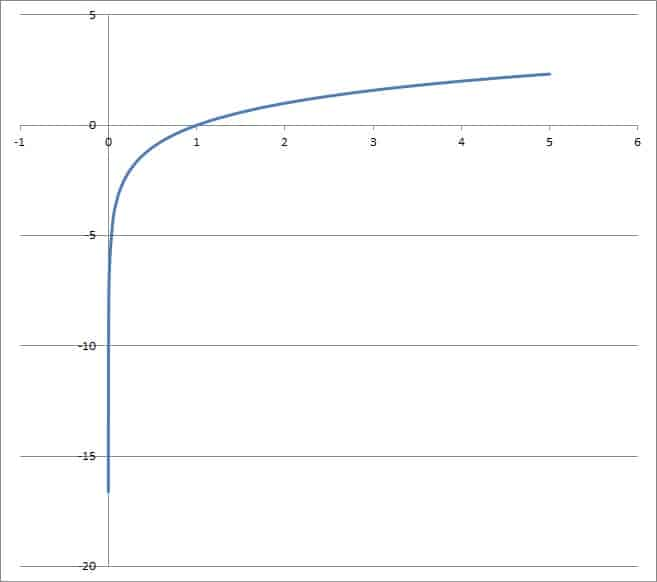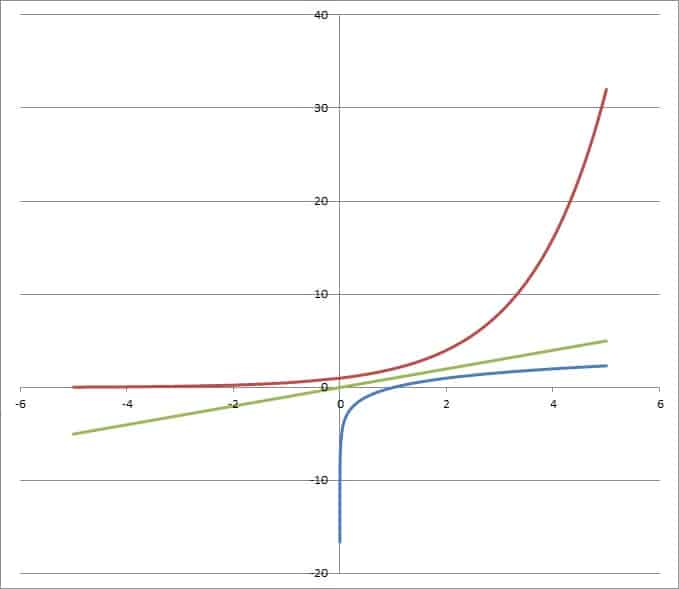Logarithms are often used in science to model things like sound (decibels), earthquake strength (Richter scale), and pH (for the strength of acids or bases).  Logarithmic functions have some interesting properties when graphed.

So, what are the most important things to remember about logarithmic functions?  The function logb(x) has no y intercept and no horizontal asymptote, but it has a vertical asymptote at x = 0.  It does not have symmetry, but it is the mirror image of its inverse function bx reflected across the line y = x.  Its domain is positive real numbers and its range is all real numbers.

Of course, we can find the inverse of a logarithmic function if we restrict its domain.  Some things (like the domain and vertical asymptote) of a logarithmic function will change if we shift it horizontally or multiply by a negative.

Let’s get started.

## 7 Common Questions About Logarithms

Logarithms are really just another way of talking about exponents.  Their graphs have some properties we can count on, although they may shift if we alter the basic logarithm function.

One tricky thing is the idea of a y intercept for a logarithmic function, so we’ll start there.

### Do Logarithmic Functions Have A Y Intercept?

The basic logarithmic function f(x) = logb(x) for real b > 0 does not have a y intercept.  Remember that a y intercept of a function happens when x = 0, but we cannot plug in x = 0 for this function (plugging in zero or negative numbers for a logarithm is undefined).

We can see this algebraically by converting from log form to exponential form and trying to solve when x = 0:

• f(x) = logb(x)  [the definition of the basic logarithmic function]
• y = logb(x)  [replace f(x) with y]
• by = x  [convert to exponential form, using log rules]
• by = 0  [substitute x = 0 to find the y intercept]

No matter which base b we are using for the logarithm, there is no value of y such that by = 0.  So, the basic logarithmic function f(x) = logb(x) has no y intercept for any real b > 0.The graph of the logarithmic function f(x) = log2(x). No matter which value of y we choose, we will never quite reach x = 0.

However, we can create a logarithmic function with a y intercept by shifting the function to the left.  For instance, let’s shift the function to the left by 1 unit.

This is easily done by replacing x by x + 1 in the function definition.

So, let’s define g(x) = logb(x + 1).  Now we can solve for the y intercept:

• g(x) = logb(x + 1)  [the definition of the modified logarithmic function]
• y = logb(x + 1)  [replace g(x) with y]
• by = x + 1  [convert to exponential form]
• by = 0 + 1  [substitute x = 0 to find the y intercept]
• by = 1
• y = 0  [b0 = 1, regardless of which b > 0 we chose for the log function]

So, the y intercept for the modified logarithmic function g(x) = logb(x + 1) is the point (0, 0).

More generally, the logarithmic function h(x) = logb(x + c) has a y intercept at the point (0, logb(c)), provided that we choose c > 0 (that is, we shift the function to the left).

On the other hand, if we shift the basic logarithmic function to the right, it will still have no y intercept.

### What Is The Domain Of A Logarithmic Function?

The domain of the basic logarithmic function f(x) = logb(x) for real b > 0 is the set of positive real numbers.

As mentioned earlier, x = 0 is undefined in this function.  However, it is also true that x < 0 is undefined, since there is no y value such that by is negative.

However, we can change the domain of a logarithmic function by it to the left or right.  For instance, let’s shift the function to the left by 1 unit again.

Once again, our modified logarithmic function is g(x) = logb(x + 1).

We can still use any positive real number x, but we can also plug in negative numbers in the set (-1, 0].  That is, we have added this set to the domain: any numbers between -1 and 0 excluding -1 but including 0.

So, the domain of the modified logarithmic function g(x) = logb(x + 1) is from -1 to infinity (excluding -1).

If we shifted the function 1 unit to the right to get logb(x – 1), the new domain would be from 1 to infinity (excluding 1).

More generally, the logarithmic function h(x) = logb(x + c) has a domain of (-c, infinity), for any value of c (positive or negative).

If we plug in –x for x, we get a logarithmic function logb(-x) with a domain of all negative numbers instead.

### What Is The Range Of A Logarithmic Function?

The range of the basic logarithmic function f(x) = logb(x) is the set of all real numbers.  We can see this by picking a y value in the range (any real number) and solving for x:

• f(x) = logb(x)  [the definition of the logarithmic function]
• y = logb(x)  [replace f(x) with y]
• by = x  [convert to exponential form]

So we just take the base b and raised it to the y power to find a value of x that will lead to the desired output.

We know that x in in the domain of the logarithmic function.  The reason is that x = by is a positive number raised to a positive number, which must also be a positive number.

Even if we shift the basic logarithmic function up or down, the range is still all real numbers.  For example, let’s define g(x) = logb(x) + d, which is the basic logarithmic function shifted up (or down) by d units (d is a real number, and it could be positive or negative).

For a given value of y, we would find the corresponding x value as follows:

• g(x) = logb(x) + d  [the definition of the modified logarithmic function]
• y = logb(x) + d  [replace f(x) with y]
• y – d = logb(x)
• by-d = x  [convert to exponential form]

Since y and d are both real numbers, then y – d is just another real number.  Then x = by-d is a positive real number, which is in the domain of the logarithmic function.

If we negate the basic log function, we get a logarithmic function -logb(x) which still has a range of all real numbers.

### Do Logarithmic Functions Have Horizontal Asymptotes?

A logarithmic function does not have a horizontal asymptote.  However, it does have a vertical asymptote (more on this shortly).

On the other hand, the inverse of a logarithmic function (which is an exponential function) will have a horizontal asymptote (but no vertical asymptote).

### Do Logarithmic Functions Have Vertical Asymptotes?

A logarithmic function has a vertical asymptote.  The basic logarithmic function f(x) = logb(x) for b > 0 has its vertical asymptote at x = 0.

As mentioned earlier, we cannot plug in x = 0 (or any negative value of x) due to the domain of the basic logarithmic function.

However, as we approach x = 0 from the right (using smaller and smaller positive x values), we get larger and larger y values.

The table below helps to illustrate using b = 10, the function g(x) = log10(x).

As you can see from the table, we have a vertical asymptote at x = 0.

If you shift the basic log function horizontally by c units, you also shift the vertical asymptote horizontally by c units.

That is, the function h(x) = logb(x – c) has a vertical asymptote at x = c (for any real value of c).

### Do Logarithmic Functions Have Symmetry?

A logarithmic function itself does not have symmetry.  However, there is some symmetry to be found if you consider a logarithmic function f(x) = logb(x) and its inverse function f-1(x) = bx (the exponential function with a base of b).

After graphing both of these functions, we can see that they are mirror images if we reflect one of them across the line y = x.

To illustrate, you can see the graph of f(x) = log2(x) and f-1(x) = 2x below.Here we have the graph of the logarithmic function f(x) = log2(x) [bottom function, in blue] and the exponential function f-1(x) = 2x [top function, in red]. Reflecting one function across the line y = x [middle line, green] gives us the other function.

### How To Find The Inverse Of A Logarithmic Function

To find the inverse of a logarithmic function, take the usual steps:

• 1. Replace f(x) by y.
• 2. Solve the equation for x.
• 3. Change y to x, and change x to f-1(x).

Let’s work through an example to see how this works in practice.

#### Example 1: How To Find The Inverse Of A Logarithmic Function

Let’s find the inverse of the logarithmic function f(x) = log2(x).

First, replace f(x) by y to get:

• y = log2(x)

Next, solve for x:

• 2y = x  [switch from log form to exponential form]

Finally, change y to x, and change x to f-1(x):

• x = 2y
• f-1(x) = 2x

So, the inverse of the logarithmic function f(x) = log2(x) is f-1(x) = 2x.

#### Example 2: How To Find The Inverse Of A Logarithmic Function

Let’s find the inverse of the logarithmic function f(x) = log3(x – 4) + 5.

First, replace f(x) by y to get:

• y = log3(x – 4) + 5

Next, solve for x:

• y = log3(x – 4) + 5
• y – 5 = log3(x – 4)  [subtract 5 from both sides]
• 3y – 5 = x – 4  [switch from log form to exponential form]
• 3y – 5 + 4 = x  [add 4 to both sides]

Finally, change y to x, and change x to f-1(x):

• x = 3y – 5 + 4
• f-1(x) = 3x – 5 + 4

So, the inverse of f(x) = log3(x – 4) + 5 is f-1(x) = 3x – 5 + 4.

## Conclusion

Now you know the answers to some common questions about logarithmic functions.  You also know how changes to the inputs will alter the domain and vertical asymptotes.